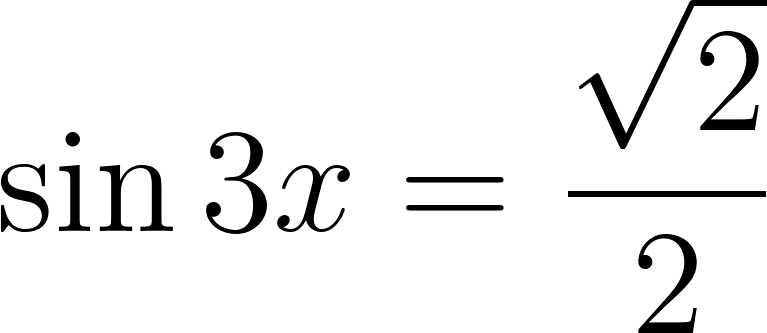×
Get Full Access to Calculus: Early Transcendentals - 1 Edition - Chapter 1.4 - Problem 32e
Get Full Access to Calculus: Early Transcendentals - 1 Edition - Chapter 1.4 - Problem 32e

×

# Ch 1.4 - 32EISBN: 9780321570567 2

## Solution for problem 32E Chapter 1.4

Calculus: Early Transcendentals | 1st Edition

• Textbook Solutions
• 2901 Step-by-step solutions solved by professors and subject experts
• Get 24/7 help from StudySoup virtual teaching assistantsCalculus: Early Transcendentals | 1st Edition

4 5 1 250 Reviews
23
4
Problem 32E

Solving trigonometric equations :Solve the equationStep-by-Step Solution:

Step 1 of 6

We need to solve the equation,Step 2 of 6

Step 3 of 6

##### ISBN: 9780321570567

The answer to “?Solving trigonometric equations :Solve the equation” is broken down into a number of easy to follow steps, and 6 words. Calculus: Early Transcendentals was written by and is associated to the ISBN: 9780321570567. This full solution covers the following key subjects: . This expansive textbook survival guide covers 112 chapters, and 7700 solutions. The full step-by-step solution to problem: 32E from chapter: 1.4 was answered by , our top Calculus solution expert on 03/03/17, 03:45PM. Since the solution to 32E from 1.4 chapter was answered, more than 418 students have viewed the full step-by-step answer. This textbook survival guide was created for the textbook: Calculus: Early Transcendentals, edition: 1.

## Discover and learn what students are asking

Calculus: Early Transcendental Functions : Conics, Parametric Equations, and Polar Coordinates
?In Exercises 1–6, match the equation with the correct graph. [The graphs are labeled (a), (b), (c), (d), (e), and (f).]

Calculus: Early Transcendental Functions : Slope Fields and Eulers Method
?In Exercises 1-8, verify the solution of the differential equation. Solution D

Unlock Textbook Solution